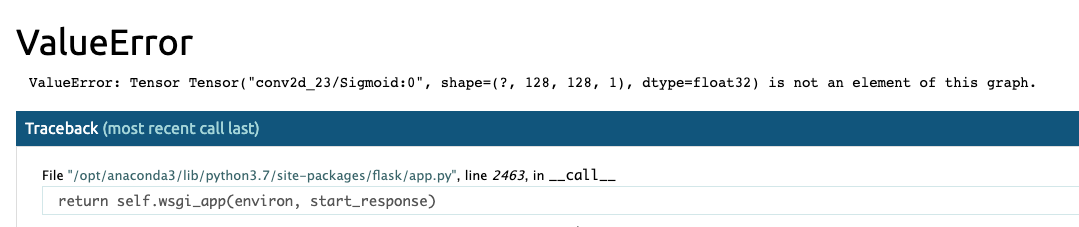2021/01/24

## 前言``````ValueError: Tensor Tensor(“xxx:0”, shape=(), dtype=float32) is not an element of this graph.
``````

## 方法一

``````import tensorflow as tf
from tensorflow.keras.backend import set_session

sess = tf.Session()
graph = tf.compat.v1.get_default_graph()
set_session(sess)
# 先載入是訓練的模型

# 在 API  Router 中每次請求模型預測時
global sess
global graph
with graph.as_default():
set_session(sess)
model.predict()
``````

## 方法二 (Class寫法)

``````import tensorflow as tf
from tensorflow.keras.backend import set_session

class MyModel():
def __init__(self):
# init backend graph
self.sess = tf.Session()
self.GRAPH = tf.compat.v1.get_default_graph()
set_session(self.sess)

def inference(self, input):
# run model
with self.GRAPH.as_default():
set_session(self.sess)
model.predict()

myModel = MyModel()
result = myModel.inference(input)
``````https://www.youtube.com/channel/UCSNPCGvMYEV-yIXAVt3FA5A